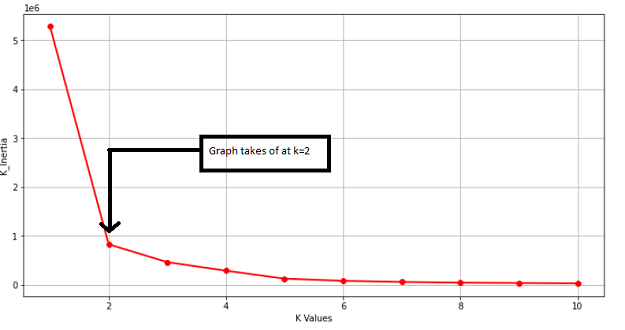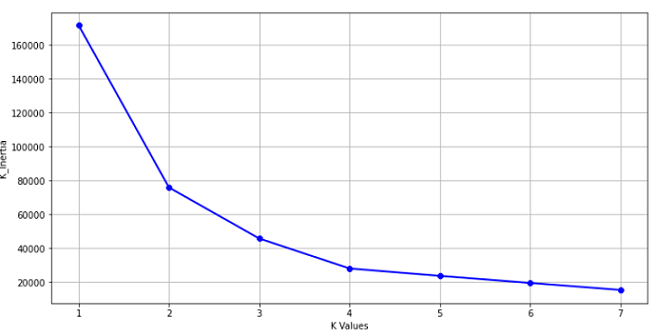Mohammed Muzammil — Published On July 27, 2021

This article was published as a part of the Data Science Blogathon

## Overview

This article will help us understand the working behind K-means Clustering Algorithm. We will look at the Euclidean Distance Formula and with the help of the formula, we will understand how clusters and centroids within the cluster move.

## Introduction

As organizations are moving forward with data-driven decisions. They depend on the available historical data to study the behaviour of the individual and understand the pattern. E-commerce, Banking, and Insurance companies are good examples of this.

Most of the Businesses mentioned above make use of the concept called Clustering. Clustering as the name describes is nothing but a grouping. Now the million-dollar question is how does it work.

1. What is Clustering?
2. Types of Clustering
3. What is K-Means Clustering
4. The flow of K-Means Algorithm
5. Understanding the working behind K-Means
6. Euclidean Distance Formula
7. Midpoint Formula to Calculate the New Centroid.
8. Implementing K-Means in Python
9. Conclusion

## 1. What is Clustering?

Clustering as a term means grouping identical elements into similar groups or clusters. It can be defined as a process of breaking down the data into groups consisting of similar data points or elements.

## 2. Types of Clustering

Exclusive Clustering – Two or more groups or clusters which do not overlap.
Overlapping Clustering – Two or more groups or clusters that overlap.
Hierarchical Clustering – It builds the hierarchy of clusters.

## 3. What is K-Means Clustering?

K-Means algorithm uses the clustering method to group identical data points in one group and all the data points in that group share common features but are distinct when compared to data points in other groups.

Points in the same group are similar as possible.
Points in different groups are as dissimilar as possible.

K – Means Clustering falls under Unsupervised Machine Learning Algorithm and is an example of Exclusive Clustering. “K” in K – Means is the number of specified clusters.

Two ways or methods to specify the Number of Clusters in K-Means.

1. Elbow Method
2. Purpose Based

Looking at the below example for the elbow method we can see that at k=2 the Graph changes exponentially, and the point where it changes is taken as the value for the clusters.

## 4. The flow Of K-Means Algorithm

1. Input the Number of Clusters
2. Calculate the Distance of the Data Point from the Cluster Centroid
3. Assign the Data Point to the two nearest Cluster
4. Re- Calculate the Centroid
5. Repeat the process for all the Data Points

## 5. Understanding the Working behind K-Means.

Let us understand the K-Means algorithm with the help of the below table, where we have data points and will be clustering the data points into two clusters (K=2).

Initially considering Data Point 1 and Data Point 2 as initial Centroids, i.e Cluster 1 (X=121 and Y = 305) and Cluster 2 (X=147 and Y = 330).

## 6. Euclidean Distance Formula

Using Euclidean Distance Formula, the remaining data points from 3 to 33 are grouped into 2 clusters k1 and k2, depending upon their distance from the Cluster Centroids.
Here xn and yn are the other data points from 3 to 33.
Here xc and yc are the assumed centroid Cluster 1 and Cluster 2
The data point 3 is near the Cluster k2 as the value 22.25 that is the Euclidean distance is less compared to the other value which is 85.63. The data point will move to Cluster k2.

## 7. Midpoint Formula to Calculate the New Centroid.

The next thing would be to find the new value for the centroid k2 by using the midpoint equation.

Now the value for the new centroid k2 is (x, y: 142.5, 319.5) and the process is repeated for the other data points.

## 8. Implementing K-Means in Python

After studying, the above equation and the basis of Euclidean Distance. We will implement the same using Python.
Import required libraries that we use in the program to run mathematical computations and run K-means.

Columns:

Data Points
Distance
Cost

Python Code

# Importing Libraries

```import pandas as pd

import numpy as np

import matplotlib.pyplot as plt

import seaborn as sns %matplotlib inline```

Creating Dataframe using Pandas. Next is to look at the Data and understand it.

```# Creating DataFrame
#Reading the File to see the shape, type and Stastics of the Data.
df.shape
df.describe()
df.dtypes```

We can see the top 5 Values from a Data Frame and the data type for the following column’s  “Data Points” and “Distance” is an int and for the column “Cost” it is Float.

The Data has 33 Rows and 3 Column’s

Checking for missing values and dropping the “Data Points” column

```df.isnull().sum()
df.drop(columns=['Data Points'],inplace = True)

To start with modeling we will create a variable and assign the values from the data to it

```#Modelling
# Creating a variable X1 and assigning the values to it.
X1 = df.values```

Importing KMeans from Sklearn.
To find the best value for “K” Clusters, we run it in a “for” loop

```from sklearn.cluster import KMeans   #Importing KMeans from sklearn and
k_inertia = []                       # Creating a list to store the kmeans.inertia_
for k in range(1,11):
kmeans = KMeans(n_clusters=k, init='k-means++')
kmeans.fit(X1)
k_inertia.append(kmeans.inertia_)```

Visualizing from the Graph to find the “K Value” where the change in the  K-Means Inertia is negligible and that is the point where the curve shoots up.

K_means inertia is the measurement of how well the clusters have formed by calculating the distance between the Cluster Centroid and the data points in that cluster.

```plt.figure(figsize=(12,6))

plt.grid()

plt.plot(range(1,11),k_inertia,linewidth = 2, color = "red", marker = "8")

plt.xlabel('K Values')

plt.ylabel("K_Inertia")

plt.show()```
``

Taking the value of K as 2 that is k = 2 we will fit “kmeans” to the data stored in the Variable X1

`kmeans = kmeans(n_clusters=2)`
```label = kmeans.fit_predict(X1)
print(label)  # To read the cordinates or values of the Cluster Centroids```

The below code gives the coordinates of the Cluster centres.

`print(kmeans.cluster_centers_)  # To plot the visualize the Data Points and the Cluster Centroids.`

The scatter plot from matplotlib plots the points. Along the x-axis is the distance and the y-axis has the cost.

```plt.scatter(X1[:,0], X1[:,1], c=kmeans.labels_, cmap='rainbow')
plt.scatter(kmeans.cluster_centers_[:,0] ,kmeans.cluster_centers_[:,1], color = 'black')
plt.title('K-Means')
plt.xlabel('Distance')
plt.ylabel('Cost')
plt.show()```

In the above Scatter plot, the Black Dots represents the Centroid and the Purple coloured and Red Colored represents Data Points.

Using the above understanding we will implement K-means for customer segmentation to identify the clusters based on “Age” and “Spending Score”

Steps

Importing Libraries

```# Importing Libraries
import numpy as np
import pandas as pd
import matplotlib.pyplot as plt
import seaborn as sns
%matplotlib inline```

Reading its head that is the top 5 rows and its shape that is, it has 200 rows and 5 Columns. The Data has the following columns: “Customer ID”, “Gender”, “Age”, “Annual Income(k\$)”, “Spending Score(1-100)”

```df = pd.read_csv("Clustering.csv")

To check the number of columns and rows

`df.shape`

The data frame has 200 rows and 5 columns.

To check for the missing Values

`df.isnull().sum()`

To check the data types

`df.dtypes`

The data type for the following column’s “Customer ID”, “Age”, “Annual Income (k\$)” and “Spending Score (1-100)” is an int and for the column “Age” it is an Object.

Dropping the customer ID column as it is not required and checking the Head.

```df.drop(['CustomerID'],axis=1, inplace=True)

The “Customer ID” column has been dropped.

Categorizing the customers based on Age and have divided into 5 groups.

```age_18_25 = df['Age'][(df.Age >=18) & (df.Age <= 25)]
age_26_35 = df.Age[(df.Age >=26) & (df.Age <= 35)]
age_36_45 = df.Age[(df.Age >=36) & (df.Age <= 45)]
age_46_55 = df.Age[(df.Age >=46) & (df.Age <= 55)]
age_55above = df.Age[df.Age >=56]```

On the x-axis, the customer Age is presented and on the Y-axis number of customer’s are marked

```agex = ["18-25","26-35","36-45","46-55","56+"]
agey = [len(age_18_25.values),len(age_26_35.values),len(age_36_45.values),len(age_46_55.values),len(age_55above.values)]```
```plt.figure(1, figsize=(15,6))                        # Plotting the Graph

sns.barplot(x=agex, y=agey, palette = 'mako')

plt.title('Number of Customer and Ages')

plt.xlabel('Age')

plt.ylabel('Number of Customer')

plt.show()```

The same can be repeated for the “Spending score (1 – 100)” Column.

Modelling following the previous example (Segmentation based on ‘Age’ and ‘Spending Score’)
To start with modeling we will create a variable and assign the values from “Age” and “Spending Score (1-100)”  to it

`X1 = df.loc[:,['Age','Spending Score (1-100)']].values`

Importing KMeans from Sklearn.
To find the best value for “K” Clusters we are running it in a “for” loop

```from sklearn.cluster import KMeans

age_ss = []

for k in range(1,8):

kmeans = KMeans(n_clusters=k, init='k-means++')

kmeans.fit(X1)

age_ss.append(kmeans.inertia_)

Visualizing from the Graph to find the K Value where the change in the K-Means Inertia is negligible and that is the point where the curve shoots up.

plt.figure(figsize=(12,6))

plt.grid()

plt.plot(range(1,8),age_ss,linewidth = 2, color = "red", marker = "8")

plt.xlabel('K Values')

plt.ylabel("K_Inertia")

plt.show()```
``

Assigning the value of clusters as 3 that is k = 3

```kmeans = KMeans(n_clusters=3)

label = kmeans.fit_predict(X1)

Custer based on Age and Spending Score (Scatter Plot)

plt.scatter(X1[:,0], X1[:,1], c=kmeans.labels_, cmap='rainbow')

plt.scatter(kmeans.cluster_centers_[:,0] ,kmeans.cluster_centers_[:,1], color = 'black')

plt.title('Clusters Of Customers')

plt.xlabel('Age')

plt.ylabel('Spending Score(1-100)')

plt.show()```

## 9. Conclusion

Segmentation is an essential tool used by a majority of businesses to understand and categorize customers based on their spending habits, income group, age, etc.
Clustering can also be extended and used in the imaging domain to split or subdivide images according to the colour pattern, grey level, contract, etc.
For businesses like E-commerce, understanding customer segments plays a vital role in up-selling and cross-selling.
This is just the tip of the iceberg. Customer segmentation is a broad concept altogether.

Hope this helped~!